Gyroscopic Effects: Vector Aspects of Angular Momentum

• Describe the right-hand rule to find the direction of angular velocity, momentum, and torque.
• Explain the gyroscopic effect.
• Study how Earth acts like a gigantic gyroscope.

Angular momentum is a vector and, therefore, has direction as well as magnitude. Torque affects both the direction and the magnitude of angular momentum. What is the direction of the angular momentum of a rotating object like the disk in [link]? The figure shows the right-hand rule used to find the direction of both angular momentum and angular velocity. Both $\mathbf{\text{L}}$

and $\mathbf{\text{ω}}$

are vectors—each has direction and magnitude. Both can be represented by arrows. The right-hand rule defines both to be perpendicular to the plane of rotation in the direction shown. Because angular momentum is related to angular velocity by $\mathbf{\text{L}}=I\mathbf{\text{ω}}$

, the direction of $\mathbf{\text{L}}$

is the same as the direction of $\mathbf{\text{ω}}$

. Notice in the figure that both point along the axis of rotation.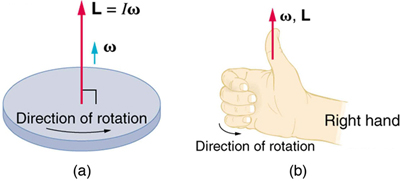Now, recall that torque changes angular momentum as expressed by

$\text{net}\phantom{\rule{0.25em}{0ex}}\mathbf{\text{τ}}=\frac{\text{Δ}\mathbf{\text{L}}}{\text{Δ}t}.$

This equation means that the direction of $\text{Δ}\mathbf{\text{L}}$

is the same as the direction of the torque $\mathbf{\text{τ}}$

that creates it. This result is illustrated in [link], which shows the direction of torque and the angular momentum it creates.

Let us now consider a bicycle wheel with a couple of handles attached to it, as shown in [link]. (This device is popular in demonstrations among physicists, because it does unexpected things.) With the wheel rotating as shown, its angular momentum is to the woman's left. Suppose the person holding the wheel tries to rotate it as in the figure. Her natural expectation is that the wheel will rotate in the direction she pushes it—but what happens is quite different. The forces exerted create a torque that is horizontal toward the person, as shown in [link](a). This torque creates a change in angular momentum $\mathbf{\text{L}}$

in the same direction, perpendicular to the original angular momentum $\mathbf{\text{L}}$

, thus changing the direction of $\mathbf{\text{L}}$

but not the magnitude of $\mathbf{\text{L}}$

. [link] shows how $\text{Δ}\mathbf{\text{L}}$

and $\mathbf{\text{L}}$

add, giving a new angular momentum with direction that is inclined more toward the person than before. The axis of the wheel has thus moved perpendicular to the forces exerted on it, instead of in the expected direction.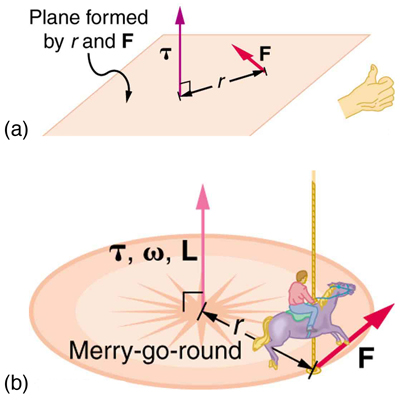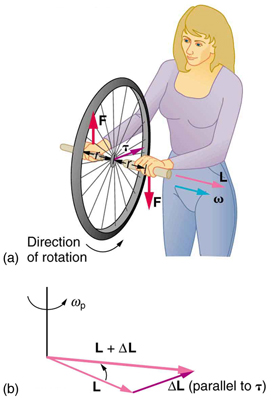This same logic explains the behavior of gyroscopes. [link] shows the two forces acting on a spinning gyroscope. The torque produced is perpendicular to the angular momentum, thus the direction of the torque is changed, but not its magnitude. The gyroscope precesses around a vertical axis, since the torque is always horizontal and perpendicular to $\mathbf{\text{L}}$

. If the gyroscope is not spinning, it acquires angular momentum in the direction of the torque ($\mathbf{\text{L}}=\text{Δ}\mathbf{\text{L}}$

), and it rotates around a horizontal axis, falling over just as we would expect.

Earth itself acts like a gigantic gyroscope. Its angular momentum is along its axis and points at Polaris, the North Star. But Earth is slowly precessing (once in about 26,000 years) due to the torque of the Sun and the Moon on its nonspherical shape.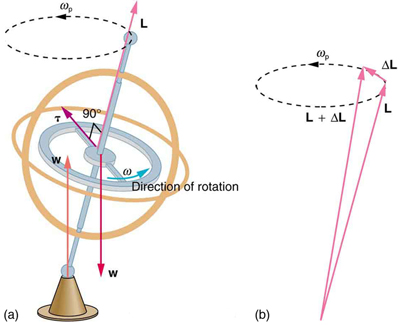Rotational kinetic energy is associated with angular momentum? Does that mean that rotational kinetic energy is a vector?

No, energy is always a scalar whether motion is involved or not. No form of energy has a direction in space and you can see that rotational kinetic energy does not depend on the direction of motion just as linear kinetic energy is independent of the direction of motion.

Section Summary

• Torque is perpendicular to the plane formed by $r$

and

$\mathbf{\text{F}}$

and is the direction your right thumb would point if you curled the fingers of your right hand in the direction of

$\mathbf{\text{F}}$

. The direction of the torque is thus the same as that of the angular momentum it produces.

• The gyroscope precesses around a vertical axis, since the torque is always horizontal and perpendicular to $\mathbf{\text{L}}$

. If the gyroscope is not spinning, it acquires angular momentum in the direction of the torque (

$\mathbf{\text{L}}=\text{Δ}\mathbf{\text{L}}$

), and it rotates about a horizontal axis, falling over just as we would expect.

• Earth itself acts like a gigantic gyroscope. Its angular momentum is along its axis and points at Polaris, the North Star.

Conceptual Questions

While driving his motorcycle at highway speed, a physics student notices that pulling back lightly on the right handlebar tips the cycle to the left and produces a left turn. Explain why this happens.

Gyroscopes used in guidance systems to indicate directions in space must have an angular momentum that does not change in direction. Yet they are often subjected to large forces and accelerations. How can the direction of their angular momentum be constant when they are accelerated?

Problem Exercises

Integrated Concepts

The axis of Earth makes a 23.5° angle with a direction perpendicular to the plane of Earth’s orbit. As shown in [link], this axis precesses, making one complete rotation in 25,780 y.

(a) Calculate the change in angular momentum in half this time.

(b) What is the average torque producing this change in angular momentum?

(c) If this torque were created by a single force (it is not) acting at the most effective point on the equator, what would its magnitude be?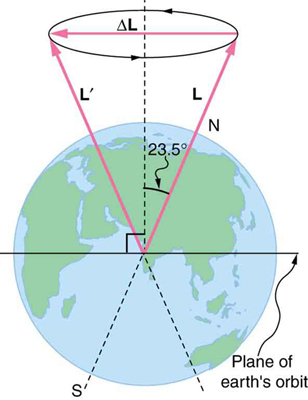(a) $5\text{.}\text{64}×{\text{10}}^{\text{33}}\phantom{\rule{0.25em}{0ex}}\text{kg}\cdot {\text{m}}^{2}\text{/s}$

(b) $1\text{.}\text{39}×{\text{10}}^{\text{22}}\phantom{\rule{0.25em}{0ex}}\text{N}\cdot \text{m}$

(c) $2\text{.}\text{17}×{\text{10}}^{\text{15}}\phantom{\rule{0.25em}{0ex}}\text{N}$

Glossary

right-hand rule
direction of angular velocity ω and angular momentum L in which the thumb of your right hand points when you curl your fingers in the direction of the disk’s rotation